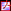Navigation: Home ›  The model ›  Special features ›  Spatial interpolation of meteorologic data ›  Combination of gradients and residuals (bilinear or spline)

# Combination of gradients and residuals (bilinear or bi-cubic spline)

A linear combination of two independent interpolations (gradients or lapse rates and residuals) is possible for results of bilinear and of bi-cubic spline interpolation methods. This is recommended if there are strong impacts of topography on the meteorologic data. Gradients are lapse rates of e.g. air temperatures. The final result is the combination of the interpolated residual (which is for example a temperature reduced to sea level) plus the value of the interpolated laspe rate multiplied with the actual elevation of the cell:
Th = T0 + h * g
where: Th = temperature at elevation h,
T0 = temperature at sea level,
h = elevation of the target cell and
g = interpolated lapse rate

Both interpolations (altitude dependent lapse rates and residuals) will be done separately using two input files provided inWaSiM table format.

This method is especially useful for using gridded climate models outputs as input for WaSiM. More details can be found in the manual in the chapter on "Interpolation of meteorologic Input Data".

BIGRES-Methode
(Bilinear Interpolation of Gradients and RESiduals)

SIGRES-Methode
(bicubic SPLINE Interpolation of Gradients and RESiduals)# WaSiM-News

05/15/2020

new release availabe:
WaSiM 10.06.00 -> More »